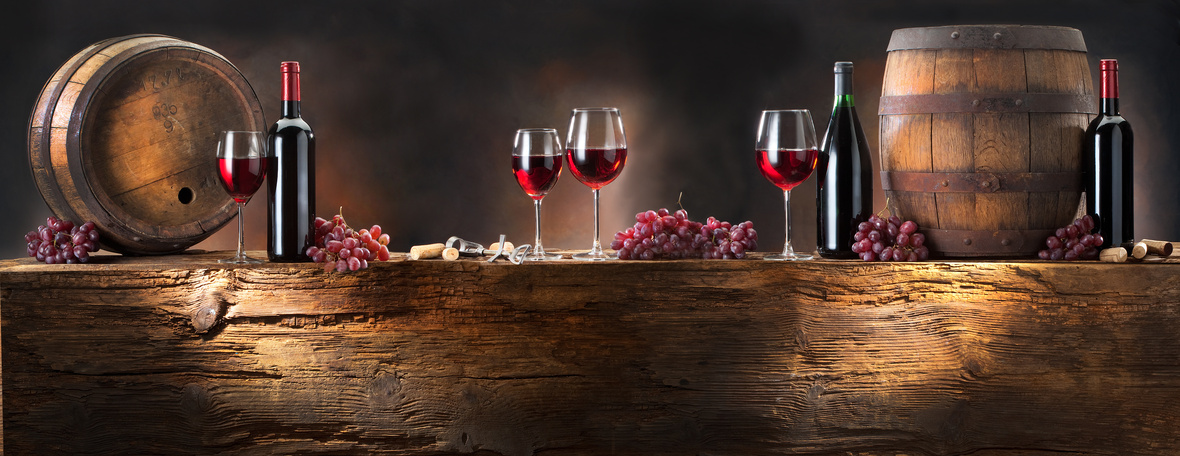# Celebrations, Contests, and Cocktails!

 /* styles */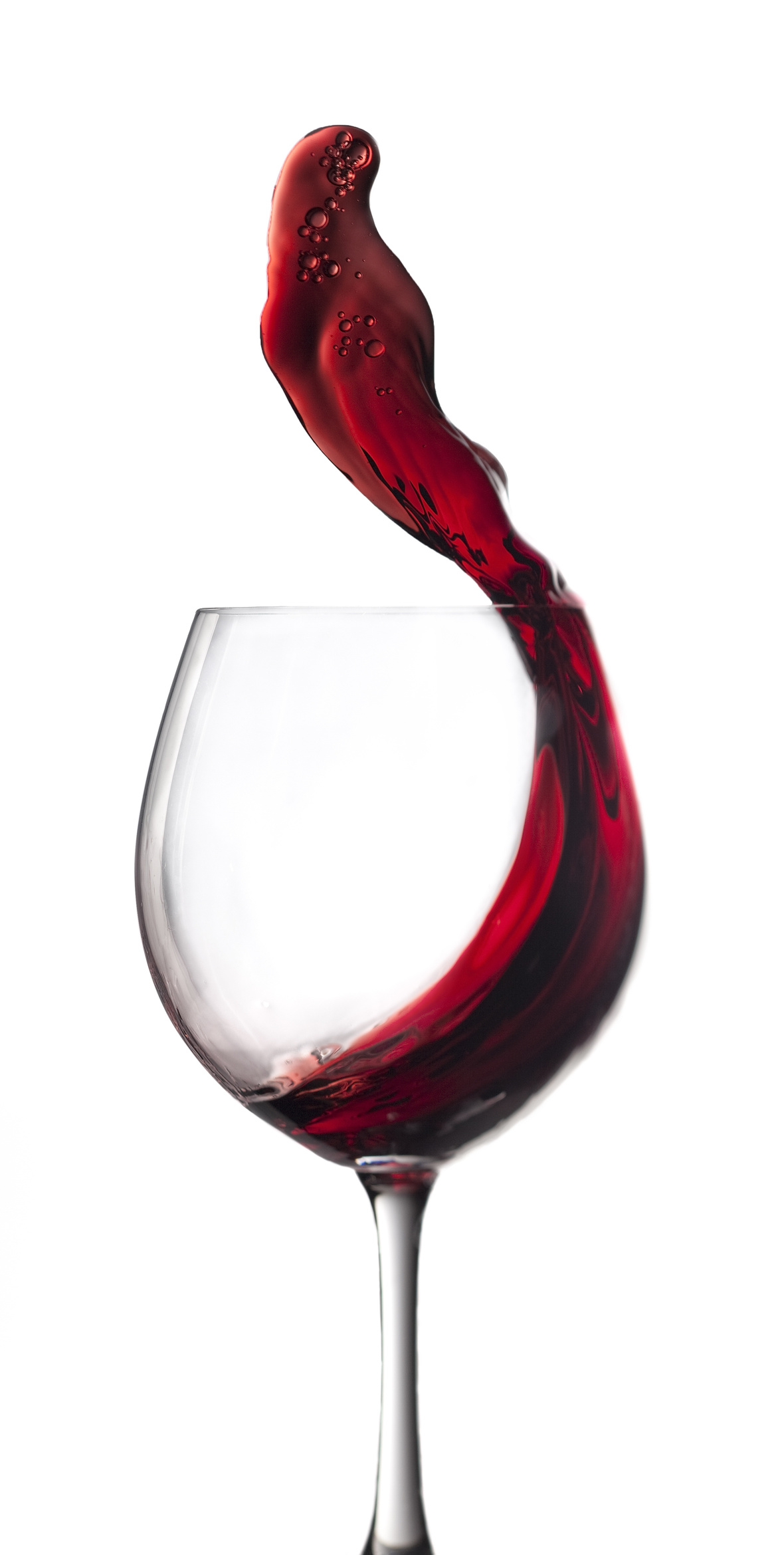Hi Wine & Spirits Lover,

The Shall We Wine team is excited to share our monthly newsletter. Our goal is to introduce you to our favorite selections, producers, distillers, varietals, regions and places to purchase and drink fabulous wine and spirits!

Cheers,

The Shall We Wine Team

 /* styles */
 table div table+table+table+table+table+table div table{width:100%;padding:0}table div table+table+table+table+table+table div table img{width:96.23%;padding:0;float:none}table div table+table+table+table+table+table div table td{width:100%;padding:0 1.88% 18px}/* styles */## THE EVOLVING LANGUAGE OF WINE

 /* styles */ Honored to have been a panelist for the 2021 Wine Writers Symposium! Check out the Jancis Robinson article on our discussion here.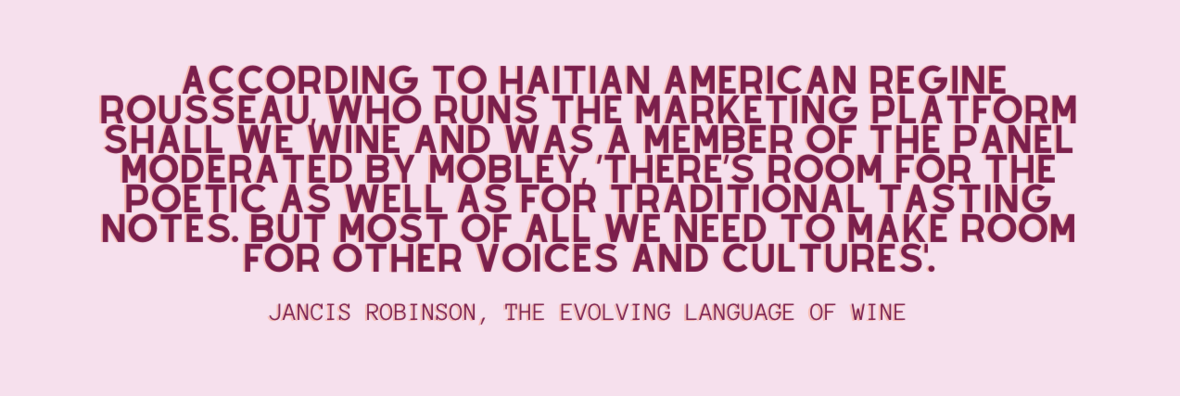table div table+table+table+table+table+table+table+table+table+table div table{width:100%;padding:0}table div table+table+table+table+table+table+table+table+table+table div table img{width:96.23%;padding:0;float:none}table div table+table+table+table+table+table+table+table+table+table div table td{width:100%;padding:0 1.88% 18px}/* styles */## SAY IT WITH PRIDEPride is more than a month-long celebration, more than a flag, more than a parade.

At SWW we have an everyday commitment and responsibility to our communities to LOVE. To love each individual, no matter race, color, religion, sex (including but not limited to gender identity and sexual orientation), and all in-between.

This means we not only acknowledge the significance of Pride, but CELEBRATE those who identify, those who have given back and those who have provided services to help these exact communities thrive.

 table div table+table+table+table+table+table+table+table+table+table+table+table+table+table div table{width:100%;padding:0}table div table+table+table+table+table+table+table+table+table+table+table+table+table+table div table img{width:96.23%;padding:0;float:none}table div table+table+table+table+table+table+table+table+table+table+table+table+table+table div table td{width:100%;padding:0 1.88% 18px}/* styles */## WIN A RIOJA SUMMER PACKAGEYou are invited to Summer Sipping with Rioja where we will be tasting Rioja White and Red Wines with Ana Fabiano, DOCa Rioja Advocacy Ambassador. Purchase your bottles and join us on IG Live Tuesday, June 22.

We will be tasting:
- EL COTO WHITE 2020
- LA ANTIGUA CLÁSICO BLANCO 2014

The Contest:

All those who FOLLOW @anafabianorioja + @riojawine (on Instagram) and tune into the Summer Sipping with Rioja Live will be entered to win the perfect Rioja Summer Package. This includes an autographed copy of The Wine Region of Rioja, a 100% cotton bar towel, and a professional wine opener. The perfect essentials to begin your summer!

Want to enter? Here are the rules:

2️ Tune into Sipping with Rioja on June 22 at 6pm CST/7pm EST on IG Live
3️ BONUS ENTRY:Tag two friends in the comments here that you would share a glass with!

GOOD LUCK!

This giveaway will close on June 22nd after the live ends, and the winner will be announced on Wednesday, June 23rd. This is not sponsored, endorsed, associated, or administered by Instagram. The winner will be chosen by a random generator. The giveaway is open to US Residents age 21 and older.

 table div table+table+table+table+table+table+table+table+table+table+table+table+table+table+table+table+table div table{width:100%;padding:0}table div table+table+table+table+table+table+table+table+table+table+table+table+table+table+table+table+table div table img{width:96.23%;padding:0;float:none}table div table+table+table+table+table+table+table+table+table+table+table+table+table+table+table+table+table div table td{width:100%;padding:0 1.88% 18px}/* styles */## SHALL WE WINE SIPS/* styles */ Are you following Shall We Wine on IG? Level up your wine game and check out our weekly series, SHALL WE WINE SIPS Tuesdays at 6:00 PM CST where we interview some of our fave people in food and wine!
 table div table+table+table+table+table+table+table+table+table+table+table+table+table+table+table+table+table+table+table+table+table div table{width:100%;padding:0}table div table+table+table+table+table+table+table+table+table+table+table+table+table+table+table+table+table+table+table+table+table div table img{width:96.23%;padding:0;float:none}table div table+table+table+table+table+table+table+table+table+table+table+table+table+table+table+table+table+table+table+table+table div table td{width:100%;padding:0 1.88% 18px}/* styles */## 2021 WINE EDUCATION SERIES WITH SWW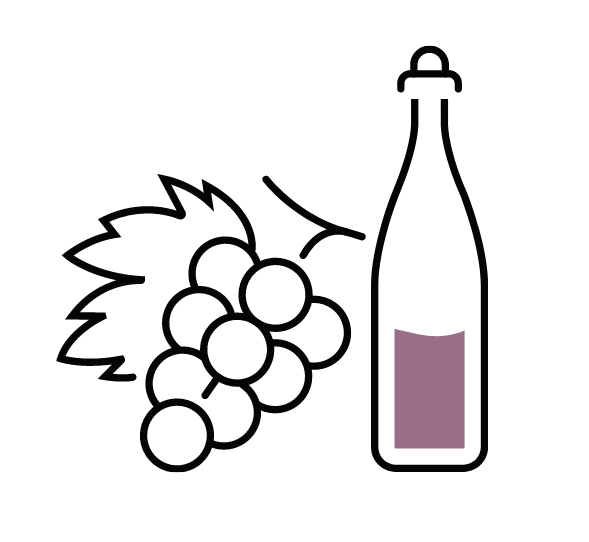Join Regine T. Rousseau, Founder and CEO for more of the amazing line-up of educational events in 2021!

See the list below, and click to reserve your spot.

Want to level up your wine drinking style without the extra fluff? Shall We Wine is a resource that helps translate complex terminology into language that individuals of every level of wine & spirits proficiency can appreciate… all while having fun!

The goal of these classes is to empower you to find the wines that fit your palate, the foods you enjoy and your mood. If you enjoy wine and are looking to deepen your knowledge of wine styles, grapes and regions, these are the classes for you!

 table div table+table+table+table+table+table+table+table+table+table+table+table+table+table+table+table+table+table+table+table+table+table+table+table div table{width:100%;padding:0}table div table+table+table+table+table+table+table+table+table+table+table+table+table+table+table+table+table+table+table+table+table+table+table+table div table img{width:96.23%;padding:0;float:none}table div table+table+table+table+table+table+table+table+table+table+table+table+table+table+table+table+table+table+table+table+table+table+table+table div table td{width:100%;padding:0 1.88% 18px}/* styles *//* styles */table div table+table+table+table+table+table+table+table+table+table+table+table+table+table+table+table+table+table+table+table+table+table+table+table+table+table+table div table{width:100%;padding:0}table div table+table+table+table+table+table+table+table+table+table+table+table+table+table+table+table+table+table+table+table+table+table+table+table+table+table+table div table img{width:96.23%;padding:0;float:none}table div table+table+table+table+table+table+table+table+table+table+table+table+table+table+table+table+table+table+table+table+table+table+table+table+table+table+table div table td{width:100%;padding:0 1.88% 18px}/* styles */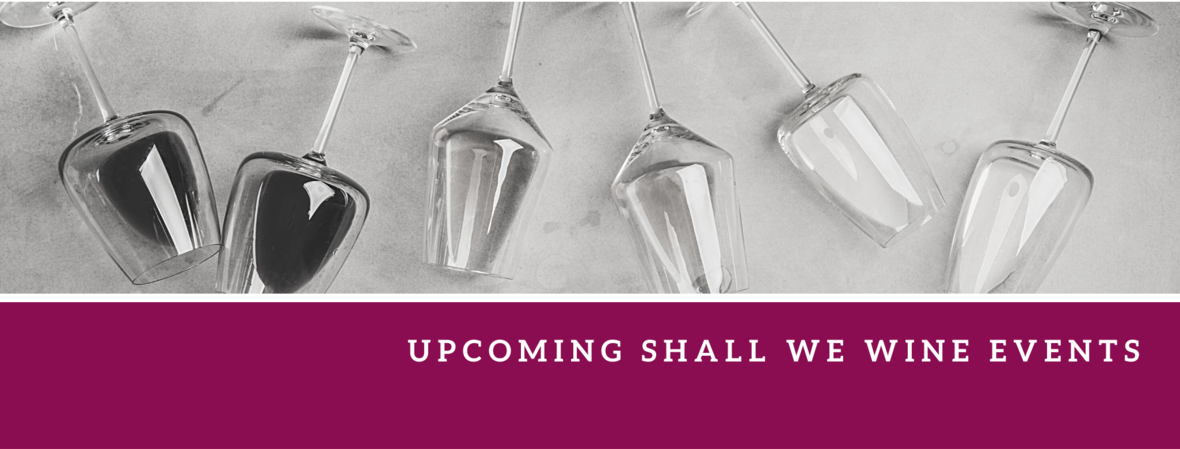/* styles */ Check out our complete events calendar to see what your next pour should be!table div table+table+table+table+table+table+table+table+table+table+table+table+table+table+table+table+table+table+table+table+table+table+table+table+table+table+table+table+table+table+table+table+table div table{width:100%;padding:0}table div table+table+table+table+table+table+table+table+table+table+table+table+table+table+table+table+table+table+table+table+table+table+table+table+table+table+table+table+table+table+table+table+table div table img{width:96.23%;padding:0;float:none}table div table+table+table+table+table+table+table+table+table+table+table+table+table+table+table+table+table+table+table+table+table+table+table+table+table+table+table+table+table+table+table+table+table div table td{width:100%;padding:0 1.88% 18px}/* styles */## WINE WITH PURPOSE: #ABottleForTheArts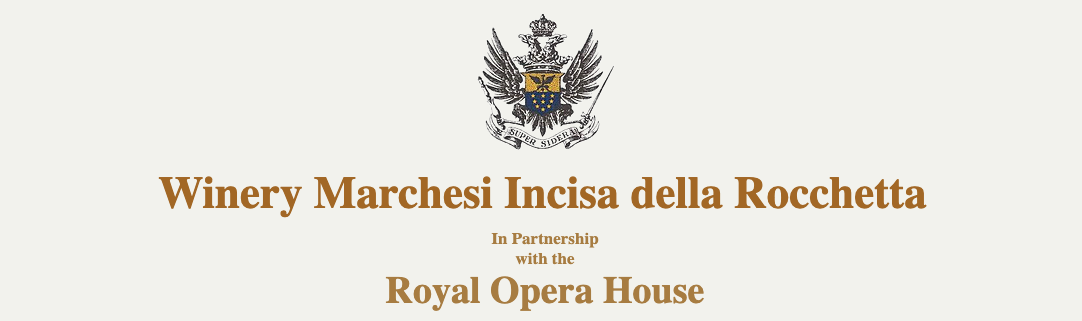/* styles */ A fascinating project that brings together wine and ballet. Marchesi Incisa della Rocchetta launches a Limited Edition label to commemorate their partnership with the Royal Opera House. Since closing its doors due to the global pandemic in March 2020, the Royal Opera House has continued to bring together the world's most extraordinary artists and curated the #OurHouseToYourHouse program. Considering this difficulty that the world of art is going through, and in particular theater and ballet, a collaboration with the Royal Opera House in London was born. An ideal and exclusive gift for lovers of the Arts. For each bottle sold, the winery will donate a portion of the profits to the Royal Opera House. This Limited Edition label will be produced in 1,000 Magnum bottles and 1,000 cases of six bottles each. Check out this video to learn more about this unique project!
 table div table+table+table+table+table+table+table+table+table+table+table+table+table+table+table+table+table+table+table+table+table+table+table+table+table+table+table+table+table+table+table+table+table+table+table+table+table div table{width:100%;padding:0}table div table+table+table+table+table+table+table+table+table+table+table+table+table+table+table+table+table+table+table+table+table+table+table+table+table+table+table+table+table+table+table+table+table+table+table+table+table div table img{width:96.23%;padding:0;float:none}table div table+table+table+table+table+table+table+table+table+table+table+table+table+table+table+table+table+table+table+table+table+table+table+table+table+table+table+table+table+table+table+table+table+table+table+table+table div table td{width:100%;padding:0 1.88% 18px}/* styles */## CAVA ROUNDUP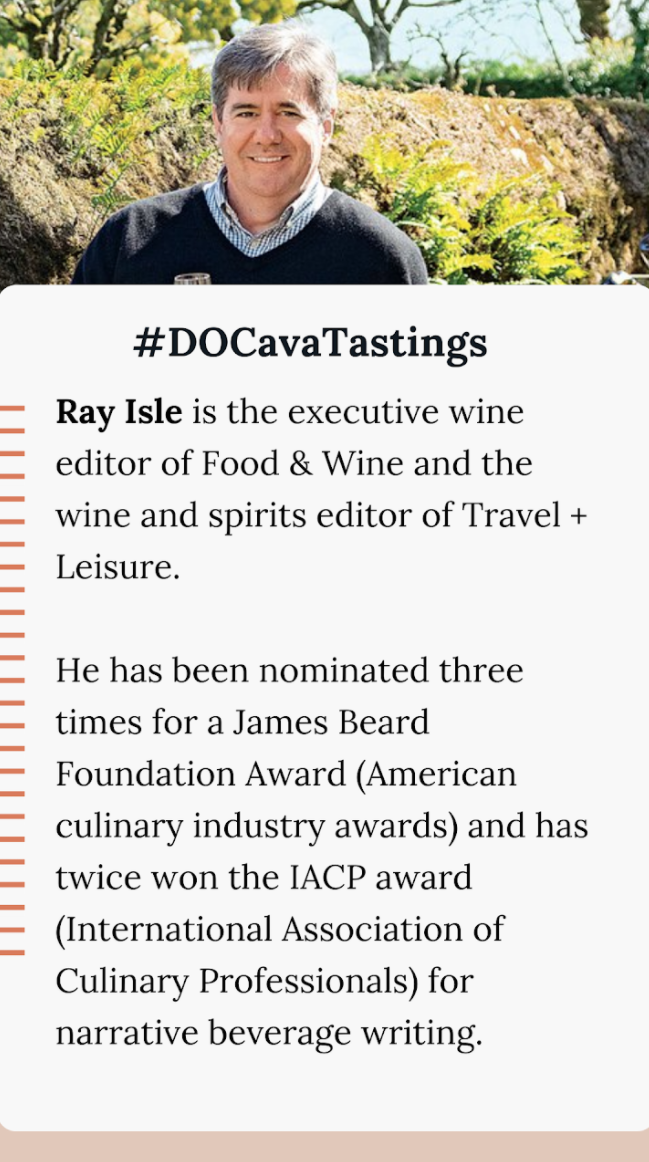Cava, the longtime darling of sommeliers and bartenders around the world, is positioning itself internationally as the quality-driven wine that offers a glass of sparkling for every occasion.

If you missed the D.O. Cava in the Kitchen Virtual Tasting with Ray Isle, executive wine editor of Food & Wine Magazine and wine and spirits editor of Travel + Leisure, earlier this month, don't fret!

Follow D.O. Cava for the latest scoop and featured events. They also host a segment called #DOCavaTalks, an interview series of influential people surrounding Cava.

If you'd like a list of the amazing wines from the event, here they are:

• Alta Alella Mirgin Rosé Reserva
• Bodegas Langa Reyes de Aragón ‘El Castro’ Reserva Brut
• Canals & Munné Dionysus Reserva Brut Nature
• Naveran Brut Vintage
• Roger Goulart Gran Reserva 2015
• Segura Viudas Brut Reserva

 table div table+table+table+table+table+table+table+table+table+table+table+table+table+table+table+table+table+table+table+table+table+table+table+table+table+table+table+table+table+table+table+table+table+table+table+table+table+table+table+table div table{width:100%;padding:0}table div table+table+table+table+table+table+table+table+table+table+table+table+table+table+table+table+table+table+table+table+table+table+table+table+table+table+table+table+table+table+table+table+table+table+table+table+table+table+table+table div table img{width:96.23%;padding:0;float:none}table div table+table+table+table+table+table+table+table+table+table+table+table+table+table+table+table+table+table+table+table+table+table+table+table+table+table+table+table+table+table+table+table+table+table+table+table+table+table+table+table div table td{width:100%;padding:0 1.88% 18px}/* styles */## FATHER'S DAY DISCOUNT OFFERWho doesn't love a discount?!

So you're stumped on what to get dad. We have a way for you to save some money on your gift. How's this for size?

Get \$30 off your first order with Wine.com.

 table div table+table+table+table+table+table+table+table+table+table+table+table+table+table+table+table+table+table+table+table+table+table+table+table+table+table+table+table+table+table+table+table+table+table+table+table+table+table+table+table+table+table+table div table{width:100%;padding:0}table div table+table+table+table+table+table+table+table+table+table+table+table+table+table+table+table+table+table+table+table+table+table+table+table+table+table+table+table+table+table+table+table+table+table+table+table+table+table+table+table+table+table+table div table img{width:96.23%;padding:0;float:none}table div table+table+table+table+table+table+table+table+table+table+table+table+table+table+table+table+table+table+table+table+table+table+table+table+table+table+table+table+table+table+table+table+table+table+table+table+table+table+table+table+table+table+table div table td{width:100%;padding:0 1.88% 18px}/* styles */## BLOG CENTRALWe have a blog! Here you will find things like our Wine of the Week and other special wine & spirits topics! Stay in the know and read up on some of the cool things we've been sharing at Shall We Wine.

Fun Topics:
(https://www.instagram.com/tv/CPou3DVgVvs/)
The Illustrious House of Champagne Billecart-Salmon
Let's Take A Peak: Ant Moore Wines
7 Steps to Reinvent Your Wine & Spirits Self

 table div table+table+table+table+table+table+table+table+table+table+table+table+table+table+table+table+table+table+table+table+table+table+table+table+table+table+table+table+table+table+table+table+table+table+table+table+table+table+table+table+table+table+table+table+table+table div table{width:100%;padding:0}table div table+table+table+table+table+table+table+table+table+table+table+table+table+table+table+table+table+table+table+table+table+table+table+table+table+table+table+table+table+table+table+table+table+table+table+table+table+table+table+table+table+table+table+table+table+table div table img{width:96.23%;padding:0;float:none}table div table+table+table+table+table+table+table+table+table+table+table+table+table+table+table+table+table+table+table+table+table+table+table+table+table+table+table+table+table+table+table+table+table+table+table+table+table+table+table+table+table+table+table+table+table+table div table td{width:100%;padding:0 1.88% 18px}/* styles */## REVISIT THE FRENCH 75Looking to mix up your cocktail routine?

Revisit the classic French 75.

Simplicity is key during these summer months and the French 75 is one yummy ticket to feeling refreshed!

The French 75 cocktail consists of gin, Champagne, lemon juice, and sugar. You'll find it comparable to another beloved cocktail, the Tom Collins, the difference being carbonated water is used instead.▪ 2 ounces dry gin
▪ ½ ounce fresh lemon juice
▪ ¼ ounce simple syrup
▪ Champagne
▪ Ice optional
 ▪ 2 ounces dry gin
 ▪ ½ ounce fresh lemon juice
 ▪ ¼ ounce simple syrup
 ▪ Champagne
 ▪ Ice optional

Combine gin, lemon juice, and simple syrup in a cocktail shaker. Fill shaker with ice, cover, and shake.

Strain into a flute. Top with Champagne, then use a lemon twist as garnish.

You can definitely tweak this to your tastes! Swap other sparkling wine options if you're putting your wallet on a diet, play around with flavored simple syrups, opt for another citrus option... whatever your heart desires! The Champagne flute is the standard drinkware for this drink, but you can definitely play with different vessels.

 table div table+table+table+table+table+table+table+table+table+table+table+table+table+table+table+table+table+table+table+table+table+table+table+table+table+table+table+table+table+table+table+table+table+table+table+table+table+table+table+table+table+table+table+table+table+table+table+table+table+table div table{width:100%;padding:0}table div table+table+table+table+table+table+table+table+table+table+table+table+table+table+table+table+table+table+table+table+table+table+table+table+table+table+table+table+table+table+table+table+table+table+table+table+table+table+table+table+table+table+table+table+table+table+table+table+table+table div table img{width:96.23%;padding:0;float:none}table div table+table+table+table+table+table+table+table+table+table+table+table+table+table+table+table+table+table+table+table+table+table+table+table+table+table+table+table+table+table+table+table+table+table+table+table+table+table+table+table+table+table+table+table+table+table+table+table+table+table div table td{width:100%;padding:0 1.88% 18px}/* styles */## ENGAGING READS FOR THE MONTH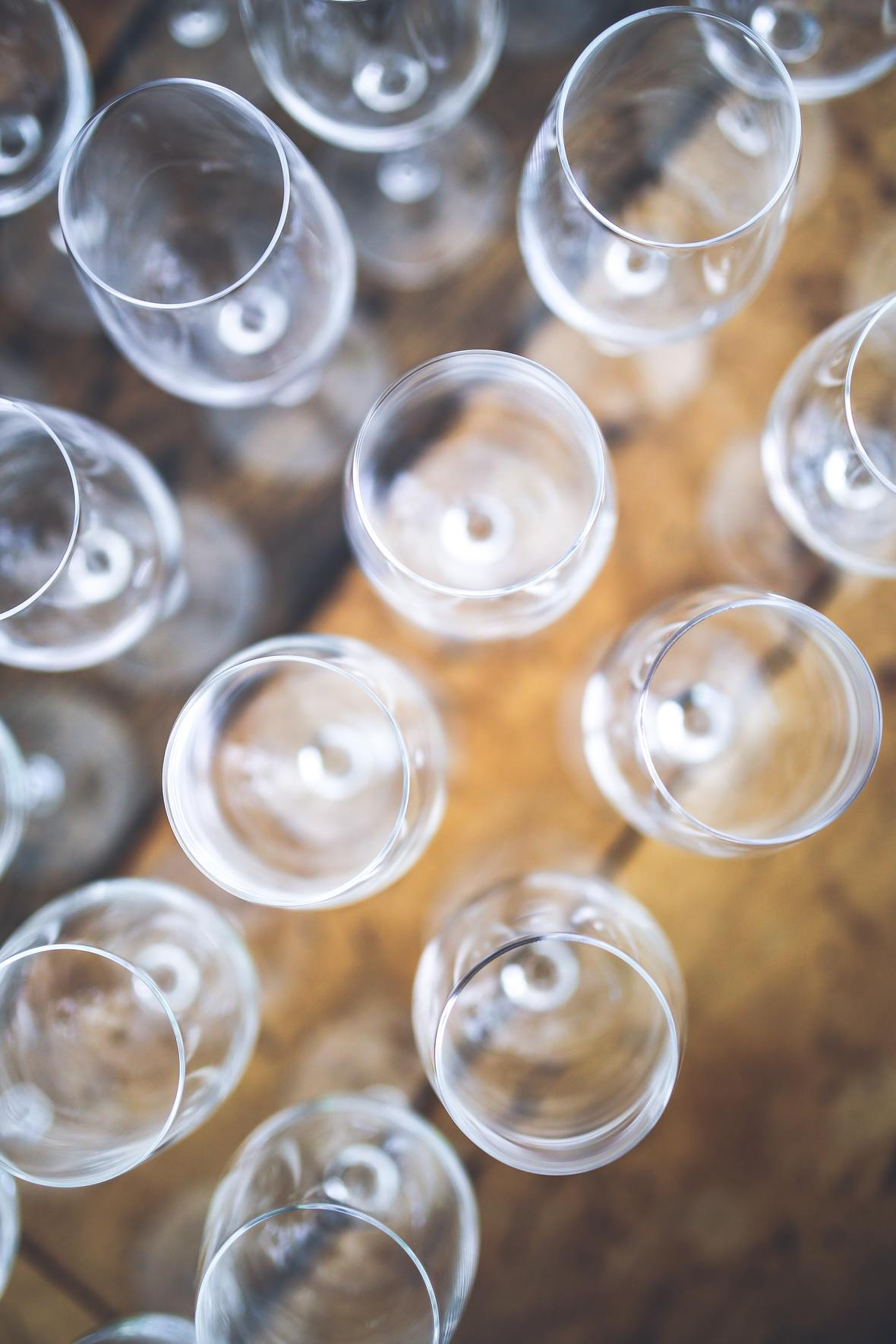Three LGBTQ+ Wine Pros Making a More Inclusive Industry
Wine Enthusiast

Why the Wine Industry Is Betting on Jermaine Stone
SevenFifty Daily

Three Ways: The Cosmopolitan
Imbibe Magazine

Scottie Pippen is Launching a Bourbon
Food & Wine

 table div table+table+table+table+table+table+table+table+table+table+table+table+table+table+table+table+table+table+table+table+table+table+table+table+table+table+table+table+table+table+table+table+table+table+table+table+table+table+table+table+table+table+table+table+table+table+table+table+table+table+table+table+table div table{width:100%;padding:0}table div table+table+table+table+table+table+table+table+table+table+table+table+table+table+table+table+table+table+table+table+table+table+table+table+table+table+table+table+table+table+table+table+table+table+table+table+table+table+table+table+table+table+table+table+table+table+table+table+table+table+table+table+table div table img{width:96.23%;padding:0;float:none}table div table+table+table+table+table+table+table+table+table+table+table+table+table+table+table+table+table+table+table+table+table+table+table+table+table+table+table+table+table+table+table+table+table+table+table+table+table+table+table+table+table+table+table+table+table+table+table+table+table+table+table+table+table div table td{width:100%;padding:0 1.88% 18px}/* styles */## STAY CONNECTED

 /* styles */ To stay in the know with the latest and greatest, make sure to follow us! Links are below.
 table div table+table+table+table+table+table+table+table+table+table+table+table+table+table+table+table+table+table+table+table+table+table+table+table+table+table+table+table+table+table+table+table+table+table+table+table+table+table+table+table+table+table+table+table+table+table+table+table+table+table+table+table+table+table+table+table div table{width:100%;padding:0}table div table+table+table+table+table+table+table+table+table+table+table+table+table+table+table+table+table+table+table+table+table+table+table+table+table+table+table+table+table+table+table+table+table+table+table+table+table+table+table+table+table+table+table+table+table+table+table+table+table+table+table+table+table+table+table+table div table table{padding:0;float:left!important;width:26.059%!important}table div table+table+table+table+table+table+table+table+table+table+table+table+table+table+table+table+table+table+table+table+table+table+table+table+table+table+table+table+table+table+table+table+table+table+table+table+table+table+table+table+table+table+table+table+table+table+table+table+table+table+table+table+table+table+table+table div table td{padding-left:29px;padding-right:29px}table div table+table+table+table+table+table+table+table+table+table+table+table+table+table+table+table+table+table+table+table+table+table+table+table+table+table+table+table+table+table+table+table+table+table+table+table+table+table+table+table+table+table+table+table+table+table+table+table+table+table+table+table+table+table+table+table div table table+table,table div table+table+table+table+table+table+table+table+table+table+table+table+table+table+table+table+table+table+table+table+table+table+table+table+table+table+table+table+table+table+table+table+table+table+table+table+table+table+table+table+table+table+table+table+table+table+table+table+table+table+table+table+table+table+table+table div table table+table+table{float:left!important;width:26.059%!important}table div table+table+table+table+table+table+table+table+table+table+table+table+table+table+table+table+table+table+table+table+table+table+table+table+table+table+table+table+table+table+table+table+table+table+table+table+table+table+table+table+table+table+table+table+table+table+table+table+table+table+table+table+table+table+table+table div table table td,table div table+table+table+table+table+table+table+table+table+table+table+table+table+table+table+table+table+table+table+table+table+table+table+table+table+table+table+table+table+table+table+table+table+table+table+table+table+table+table+table+table+table+table+table+table+table+table+table+table+table+table+table+table+table+table+table div table table+table td,table div table+table+table+table+table+table+table+table+table+table+table+table+table+table+table+table+table+table+table+table+table+table+table+table+table+table+table+table+table+table+table+table+table+table+table+table+table+table+table+table+table+table+table+table+table+table+table+table+table+table+table+table+table+table+table+table div table table+table+table td{padding-left:0;padding-right:20px}table div table+table+table+table+table+table+table+table+table+table+table+table+table+table+table+table+table+table+table+table+table+table+table+table+table+table+table+table+table+table+table+table+table+table+table+table+table+table+table+table+table+table+table+table+table+table+table+table+table+table+table+table+table+table+table+table div table table+table+table+table{float:left!important;width:21.822999999999993%!important}table div table+table+table+table+table+table+table+table+table+table+table+table+table+table+table+table+table+table+table+table+table+table+table+table+table+table+table+table+table+table+table+table+table+table+table+table+table+table+table+table+table+table+table+table+table+table+table+table+table+table+table+table+table+table+table+table div table table+table+table+table td{padding-left:0;padding-right:0}/* styles *//* styles */ Learn, Drink, Repeat! The Shall We Wine Team
 Like   Tweet   Pin   +1   in